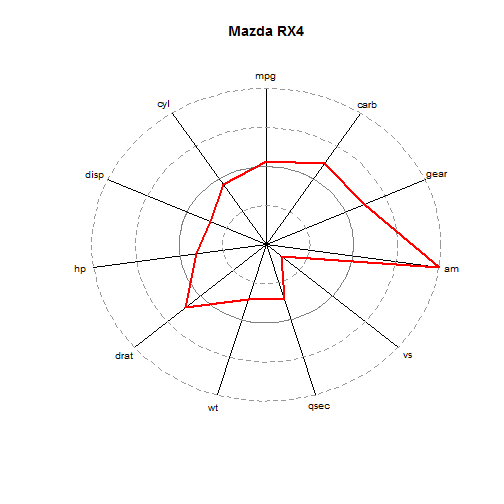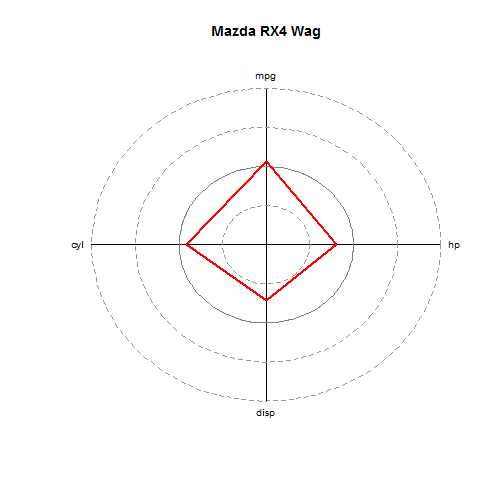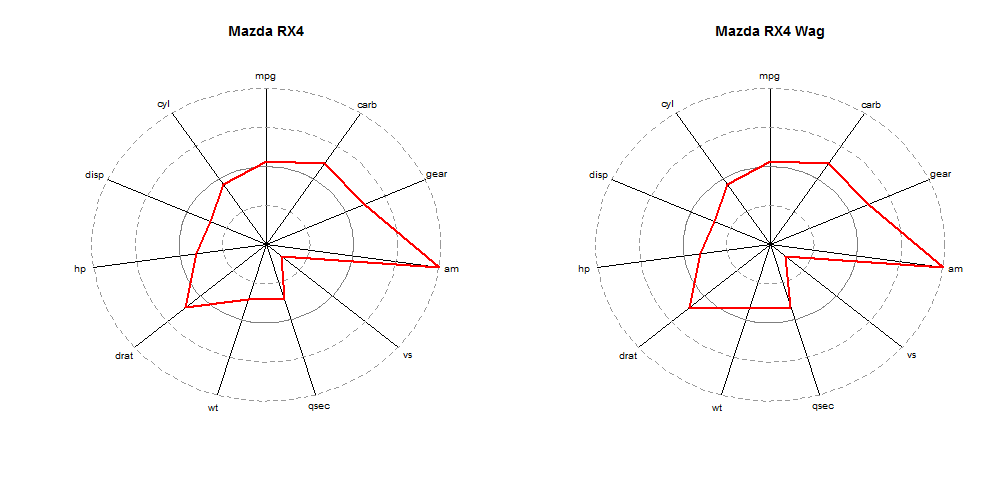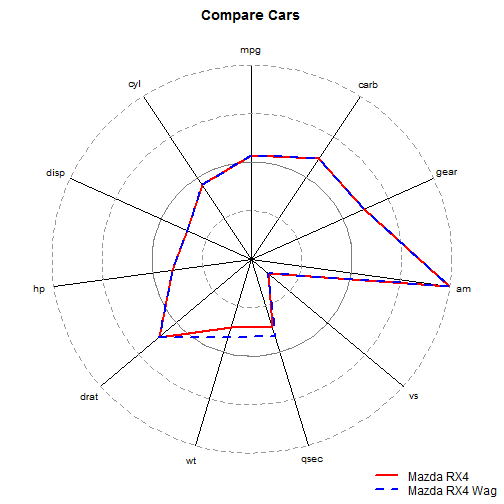## Tuesday, November 5, 2013

### Spider Web Plots in R

**Edit** I found a similar plotting technique using radarchart from the fmsb package, found at http://artax.karlin.mff.cuni.cz/r-help/library/fmsb/html/radarchart.html

Unless your brain works differently than mine, then polar coordinates aren't great for being precise in consuming and measuring data. Use bar charts, not pie charts, right angles! That being said, “spider plots” or “web plots” or whatever you want to call these charts, can succinctly summarize a lot of information, and are visually pleasing.

Here's a function to create this kind of plot.

``````
webplot = function(data, data.row = NULL, y.cols = NULL, main = NULL, add = F,
col = "red", lty = 1, scale = T) {
if (!is.matrix(data) & !is.data.frame(data))
stop("Requires matrix or data.frame")
if (is.null(y.cols))
y.cols = colnames(data)[sapply(data, is.numeric)]
if (sum(!sapply(data[, y.cols], is.numeric)) > 0) {
out = paste0("\"", colnames(data)[!sapply(data, is.numeric)], "\"",
collapse = ", ")
stop(paste0("All y.cols must be numeric\n", out, " are not numeric"))
}
if (is.null(data.row))
data.row = 1
if (is.character(data.row))
if (data.row %in% rownames(data)) {
data.row = which(rownames(data) == data.row)
} else {
stop("Invalid value for data.row:\nMust be a valid rownames(data) or row-index value")
}
if (is.null(main))
main = rownames(data)[data.row]
if (scale == T) {
data = scale(data[, y.cols])
data = apply(data, 2, function(x) x/max(abs(x)))
}
data = as.data.frame(data)
n.y = length(y.cols)
polar.vals = (90 + seq(0, 360, length.out = n.y + 1)) * pi/180

#
plot(0, xlim = c(-2.2, 2.2), ylim = c(-2.2, 2.2), type = "n", axes = F,
xlab = "", ylab = "")
title(main)
lapply(polar.vals, function(x) lines(c(0, 2 * cos(x)), c(0, 2 * sin(x))))
lapply(1:n.y, function(x) text(2.15 * cos(polar.vals[x]), 2.15 * sin(polar.vals[x]),
y.cols[x], cex = 0.8))

lapply(seq(0.5, 2, 0.5), function(x) lines(x * cos(seq(0, 2 * pi, length.out = 100)),
x * sin(seq(0, 2 * pi, length.out = 100)), lwd = 0.5, lty = 2, col = "gray60"))
lines(cos(seq(0, 2 * pi, length.out = 100)), sin(seq(0, 2 * pi, length.out = 100)),
lwd = 1.2, col = "gray50")
}

r = 1 + data[data.row, y.cols]
xs = r * cos(polar.vals)
ys = r * sin(polar.vals)
xs = c(xs, xs)
ys = c(ys, ys)

lines(xs, ys, col = col, lwd = 2, lty = lty)

}
``````

Using the mtcars data set:

``````webplot(mtcars)
``````Basically, we take a data frame, scale all numeric columns, and then plot how far above/below average (the thick black line) each of the values are for a particular row of data.

Here are the Arguments:

• data - data.frame or matrix
• data.row - row of data to plot (if NULL uses row 1)
• y.cols - columns of interest (if NULL it selects all numeric columns)
• main - title of plot (if NULL then rowname of data)
• add - whether the plot should be added to an existing plot
• col - color of the data line
• lty - lty of the data line

For example, we can change the data row that we plot, as well as the columns we want to include

``````webplot(mtcars, 2, y.cols = c("mpg", "cyl", "disp", "hp"))
``````It turns out that this isn't the easiest way to compare results, it might be helpful to overlay plots

``````par(mfcol = c(1, 2))
webplot(mtcars, "Mazda RX4")
webplot(mtcars, "Mazda RX4 Wag")
````````````par(mfcol = c(1, 1))
``````
``````par(mar = c(1, 1, 2, 1))
webplot(mtcars, "Mazda RX4", main = "Compare Cars")
webplot(mtcars, "Mazda RX4 Wag", add = T, col = "blue", lty = 2)
par(new = T)
par(mar = c(0, 0, 0, 0))
plot(0, type = "n", axes = F)
legend("bottomright", lty = c(1, 2), lwd = 2, col = c("red", "blue"), c("Mazda RX4",
"Mazda RX4 Wag"), bty = "n")
``````Which makes it easy to observe that the Mazda RX4 and Mazda RX4 Wagon are very similar, with the Wagon being a little heavier, and takes a little longer to travel a quarter mile